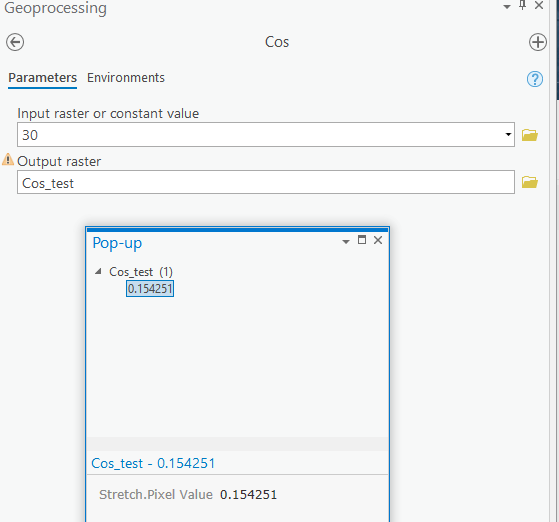# geoprocessing - Cosine 30

279
1
07-24-2019 07:11 AMNew Contributor II

Hello,

can somebody tell me why for the Math function "Cosine" of the Spatial Analyst the Cosine of 30 is 0.154251 instead of 0.8660254?

I tried to create a constant grid using the cosine function and this is the result.

Many thanks

Fabio1 Solution

Accepted SolutionsbyMVP Esteemed Contributor

angles must be in radians and not in degrees

``````import math

math.cos(30)
Out: 0.15425144988758405

Out: 0.8660254037844387‍‍‍‍‍‍‍``````byMVP Esteemed Contributor

angles must be in radians and not in degrees

``````import math

math.cos(30)
Out: 0.15425144988758405